- BetterExplained - https://betterexplained.com -

# Navigate a Grid Using Combinations And Permutations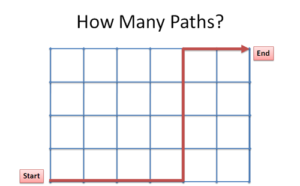Puzzles can help develop your intuition -- figuring how to navigate a grid helped me understand combinations and permutations.

Suppose you're on a 4 × 6 grid, and want to go from the bottom left to the top right. How many different paths can you take? Avoid backtracking -- you can only move right or up.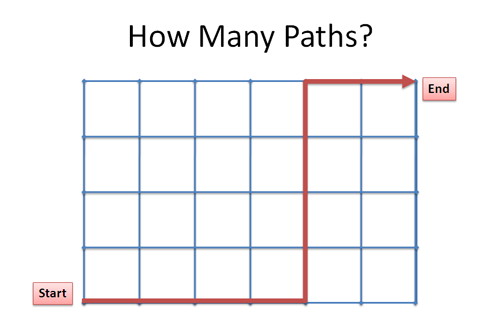Spend a few seconds thinking about how you'd figure it out.

## Insight: Convert Pictures To Text

When considering the possible paths (tracing them out with your finger), you might whisper "Up, right, up, right...".

Why not write those thoughts down? Using "u" and "r" we can write out a path:

r r r r r r r u u u u


That is, go all the way right (6 r's), then all the way up (4 u's). The path in the diagram would be:

r r r r u u u u r r


Using the text interpretation, the question becomes "How many ways can we re-arrange the letters rrrrrruuuu?"

Ah, the ubiquitous combination/permutation problem -- never thought it'd be useful, eh?

## Understanding Combinations And Permutations

There's several ways to see combination and permutation problems. Once the first explanation clicks, we can go back and see it a different way. When trying to build math intuition for a problem, I imagine several mental models circling a core idea. Starting with one insight, I work around to the others.

## Approach 1: Start The Same

Instead of having 6 rights at 4 ups, imagine we start with 10 rights (r r r r r r r r r r).

Clearly this won't do: we need to change 4 of those rights into ups. How many ways can we pick 4 rights to change?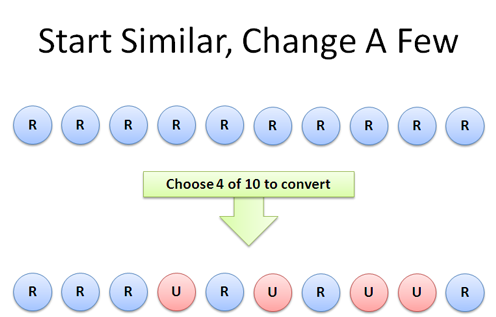Well, we have 10 choices for the first 'right' to convert (see the combinations article). And 9 for the second, 8 for the third, and 7 choices for the final right-to-up conversion. There are 10 * 9 * 8 * 7 = 10!/6! = 5040 possibilities.

But, wait! We need to remove the redundancies: after all, converting moves #1 #2 #3 and #4 (in that order) is the same as converting #4 #3 #2 #1. We have 4! (4 * 3 * 2 * 1 = 24) ways to rearrange the ups we picked, so we finally get: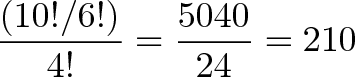$\displaystyle{\frac{(10!/6!)}{4!} = \frac{5040}{24} = 210 }$

We're just picking the items to convert (10!/6!) and dividing out the redundancies (4!).

## Approach 2: Just Use the Combination Formula

Halfway through that explanation, you might have realized we were recreating the combination formula: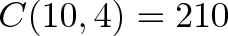$\displaystyle{C(10,4) = 210}$

That's the shortcut when you know order doesn't matter. However, sometimes I'm not sure whether I need a permutation or combination from the outset. While saying "Just use C(10,4)" may be accurate, it's not helpful as a teaching tool. Sometimes it helps to re-create the situation on your own.

## Approach 3: Start Different

Here's another approach: instead of letting each r and u be interchangeable, label the 'right' moves r1 to r6, and the 'up' moves u1 to u4. How many ways can we re-arrange these 10 items?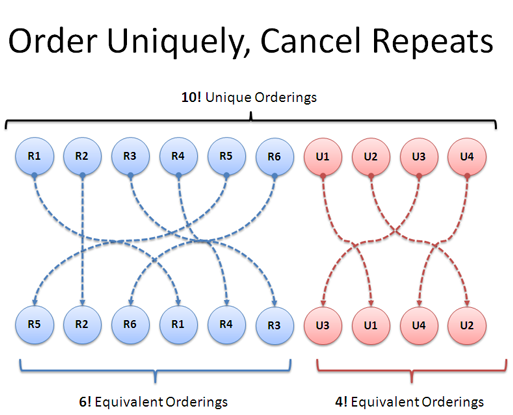This question is easy: 10! = 3,628,800 (wow, big number). We have 10 choices for the 1st move, 9 for the second, and so on, until we have 2 choices for the 9th and only 1 for the last. Cool.

Of course, we know that "r1 r2 u1 u2" is the same path as "r2 r1 u2 u1". We can shuffle the r's and u's in their own subgroups and the path will stay the same.

• How many ways can we shuffle all 10? 10! = 3,628,800
• How many ways can we shuffle 6 r's? 6! = 720
• How many ways can we shuffle 4 u's? 4! = 24

So, we start with the total number of possibilities (10! = 3,628,800) and divide out the cases where we shuffle the r's (6! = 720) and the u's (4! = 24):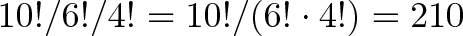$\displaystyle{10! / 6! / 4! = 10! / (6! \cdot 4!) = 210}$

Neat! It's cool seeing the same set of multiplications and divisions in different ways, just by regrouping them.

## Why is this useful?

One goal is to learn how problems can be transformed. Remember that painting of the old lady & young woman?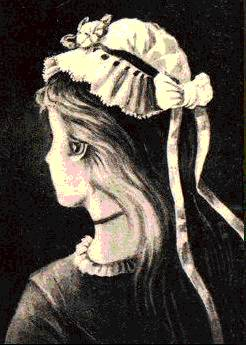Do you see both? Can you switch between them? Isn't that cool?

Part of the fun of the grid-path puzzle is seeing how to look at a problem using a visual or text metaphor. The more math you learn, the more models you have available, and you can turn problems into each other.

This doesn't have to be "practical" -- it's fun to see how listing out paths can be be done simply using letters on paper.

In math lingo, problems which can be converted to each other are "isomorphic". Mathematically, they may be the same -- but from a human perspective, one may be easier than the other (like seeing the old woman or young woman first).

For the grid puzzle, we used each perspective where comfortable:

• Visualizing the grid to understand the general problem and see a single path.
• Write the paths as text to see the general format of all paths & an easy method to enumerate them

And that's the key lesson: It's completely fine to use one model to understand the idea, and another to work out the details. Math becomes difficult when we think there's only one way to approach it.

## Variations and Extensions

Now that we've been building our mental models, let's tackle some harder problems.

Imagine your "grid" is actually in 3 dimensions. This is harder to draw, but the text representation keeps on working. Let's say we have a cube (x, y and z dimensions) that is 5 units long on each side. How many paths are there from one corner to its opposite?

Hrm. In this case, I might try the second approach, where we listed out all the possibilities. Assume we label each move differently: we have 5 uniquely-labeled moves of each type (x1-x5, y1-y5, z1-z5). We can arrange these in 15! ways (it's huge: 1.3 trillion). But, we need to remember to divide out the redundancies for each dimension.

There are 5! ways to rearrange the 5 identical motions in each direction, and we divide them out: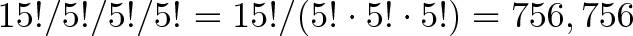$\displaystyle{15! / 5! / 5! / 5! = 15!/(5!\cdot 5!\cdot 5!) = 756,756}$

Wow, that's huge number of paths on a small cube! Earlier today you'd have trouble with the question -- I know I would have. But starting with the grid example and converting it to text, we've beefed up our model to handle 3 dimensions. Paths in four, five or 10-d should be no problem.

## Redefining The Problem

Here's the fun part: instead of changing how we see the solution, why not change the problem? What else could "Find paths on a grid" represent?

• Trap platform: Let's say you're making a set of trapdoors 4 × 6, with only 1 real path through (the others drop you into a volcano). What are the chances someone randomly walks through? With a 4×6 it's 210, as before. With a 12×12 grid it's 24!/12!12! = 2.7 million paths, with only 1 correct one.

• Order of operations: Suppose you have 10 sets of exercises to do: 4 identical leg exercises, and 6 identical arm exercises. How many different routines can you pick? This is the same as navigating the path, except the axis labels are "legs" and "arms" instead of "right" and "up".

• Random walk. Suppose we know an object moves randomly up or right. What's the chance it hits our desired endpoint after 10 steps? Well, there are 2^10 = 1024 ways to move up or right (pick "u" or "r" 10 times), and 210 ways to get to our exact destination. Therefore, you can expect to hit our spot 210 / 1024 = 20.5% of the time!

Here's a calculator to play with a few variations:

## Onward and Upward

Puzzles are a fun way to learn new mental models, and deepen your understanding for the ones you're familiar with. While I might "know" combinations and permutations, it's not until I recognize them in the wild do I feel really comfortable. Ideas do no good sitting inside your head like artifacts in a museum -- they need to be taken out and played with. Happy math.